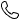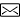0373-59399252851259250@qq.com# 基于经验模态分解的激光散斑噪声抑制方法

2 基本原理

2.1　结合EMD的Canny算子改进P-M方程法在全息散斑噪声抑制中的应用

H'(x,y)=H(x,y)N(x,y)

， （1）

（1）对图像H'(x,y)进行EMD分解，式（1）可以改写为：

H'(x,y)=(∑i=1nCi+r)N(x,y)

. （2）

H''(x,y)=C1N(x,y)

. （3）

（2）对重构的图像H''(x,y)进行Canny边缘检测，得到边缘检测的结果C(x,y)，并记录上阈值k1。

（3）根据边缘检测结果引导各向异性扩散方程的扩散程度，进行去噪，得到散斑抑制后的全息图H'''(x,y)。

（4）随后对散斑抑制后的全息图H'''(x,y)进行数值重建，从再现像中提取出相位信息进行定量相位成像。由于此时的相位信息包裹在（-π，π）之中，需要使用相应的算法进行相位展开以获得真实的相位信息。最后根据相位误差的来源，采用相对应的相位补偿算法对误差进行补偿。

2.2　EMD

（1）找出f(x,y)的极大值和极小值，通过插值法构造出极大值曲面u(x,y)和极小值曲面v(x,y)，极大值曲面和极小值曲面的均值为：

e1(x,y)=u(x,y)+v(x,y)2

. （4）

h1(x,y)=f(x,y)−e1(x,y)

. （5）

（2）将h1(x,y)作为新的输入重复上述过程k次，直到满足式（6）时方可停止循环。其中，SD的值一般在0.1~0.5之间，α一般为0.2。

SD=∑x=1X∑y=1Y∣∣h1(k−1)(x,y)−h1k(x,y)∣∣2h1k2(x,y)≤α

. （6）

（3）令r1(x,y)表示图像f(x,y)去除最高频信息C1之后剩余的部分，则有：

r1(x,y)=f(x,y)−C1

. （7）

（4）令r1(x,y)作为新的待分析图像，并重复步骤（1）和步骤（2）的计算过程之后，得到第2个IMF分量C2。

（5）重复上述过程n次，当rn(x,y)和Cn小于预定误差或者rn(x,y)为单调函数时，则不能再从图像f(x,y)中提取出IMF分量。此时，图像f(x,y)如式（8）表示：

f(x,y)=∑i=1nCi+r

. （8）

2.3　Canny算子改进P-M方程法

（1）由于梯度算子∇无法去除大的孤立噪声点，在大的噪声点处的梯度值会很大，同时扩散系数c与梯度值成反比，这会导致此处的扩散系数c的值变小，达不到扩散去噪的效果。

（2）梯度算子∇对噪声的敏感度高，抗噪性能不强，不能识别伪边缘。

∂I(x,y,t)∂t=div[(1−K) ⋅c(|∇I(x,y,t)|)⋅∇I(x,y,t)]

， （9）

c(x)=exp(−xk2)

， （10）

c(x)=11+(xk)2

， （11）

（1）对图像g(x,y)进行Canny边缘检测，得到边缘检测的结果C(x,y)，以及记录上阈值k1。

（2）按上、下、左、右4个方向求解图像g(x,y) 4个方向上的梯度，即∇N、∇S、∇E和∇W。

（3）令扩散系数c中的参数k等于上阈值k1，同时结合4个方向的梯度求解得到cN、cS、cE和cW。

（4）由于Canny边缘检测结果C(x,y)中只有0和1两种值，当C(x,y)=1时，令Canny算子K=0.01；当C(x,y)=0时，令Canny算子K=0.99。

（5）散度算子div可以按照式（12）计算：

div=14(cN×

∇N

+cS×

∇S

+cE×

∇E

+cW×

∇W)

. （12）

（6）图像g(x,y)经过Canny算子改进P-M方程法处理后，可以表示为：

g'(x,y)=g(x,y)+(1−K)div

. （13）

3 实验

3.1　实验装置及样本

Fig.1  Schematic diagram and physical diagram of digital recording optical path based on Mach-Zehnder interferometer. （a） Schematic diagram； （b） Physical diagram.

Fig.2  Samples and holograms. （a） Sample plate； （b） Sample A； （c） Sample B； （d） Sample C； （e） Sample D； （f） Sample E； （g） Hologram A corresponding to sample A； （h） Hologram B corresponding to sample B； （i） Hologram C corresponding to sample C； （j） Hologram D corresponding to sample D； （k） Hologram E corresponding to sample E.

3.2　结合EMD的Canny算子改进P-M方程法的相位重建

Fig.3  Reconstruction results with different IMF components selected. （a） Reconstructed image with C1； （b） Reconstructed image with C3； （c） Reconstructed image with C5； （d） Reconstructed image with C7.

Fig.4  Canny operator edge detection results. （a） Edge detection results of Canny operator for Fig.3（a）； （b） Edge detection results of Canny operator for Fig.2（h）.

Fig.5  Holograms processed by the proposed method. （a） Hologram A； （b） Hologram B； （c） Hologram C； （d） Hologram D； （e） Hologram E.

Fig.6  Comparison of the reconstructed phase maps after processing by the proposed method and the reconstructed phase maps without denoising holograms. （a） Reconstructed phase map of hologram A after processing by the proposed method； （b） Reconstructed phase map of hologram B after processing by the proposed method； （c） Reconstructed phase map of the hologram C after processing by the proposed method； （d） Reconstructed phase map of hologram D after processing by the proposed method； （e） Reconstructed phase map of the hologram E after processing by the proposed method； （f） Reconstructed phase map of hologram A without denoising； （g） Reconstructed phase map of hologram B without denoising； （h） Reconstructed phase map of hologram C without denoising； （i） Reconstructed phase map of hologram D without denoising； （j） Reconstructed phase map of hologram E without denoising.

3.3　实验对比

Fig.7  Reconstructed phase maps of hologram A， B， C， D and E after processing by the improved P-M equation method based on the Canny operator， mean filter method and median filter method. （a）~（e） Reconstructed phase maps after processing by the improved P-M equation method based on Canny operator； （f）~（j） Reconstructed phase maps after processing by the mean filtering method； （k）~（o） Reconstructed phase maps after processing by the median filter method.

4 分析与讨论

4.1　降噪定量分析

SSIM的计算公式如式（14）所示：

SSIM=l(x,y)⋅c(x,y)⋅s(x,y)

， （14）

EPI的计算公式如式（15）所示：

EPI=∑i=1m∑j=1n|GR11−GR21|after∑i=1m∑j=1n|GR12−GR22|before

， （15）

SSI的计算公式如式（16）所示：

SSI=var(Iafter)−−−−−−−−√mean(Iafter)⋅mean(Ibefore)var(Ibefore)−−−−−−−−√

， （16）

5种全息图经过所提出方法、基于Canny算子改进P-M方程法、均值滤波法以及中值滤波法处理之后重建相位图对应的SSIM、EPI和SSI计算结果如表1所示。

Tab.1  Calculation results of SSIM， EPI and SSI for different speckle suppression methods

4.2　相位截面曲线分析

Fig.8  Phase maps shown in Fig.6（a）， Fig.7（a）， Fig.7（f） and Fig.7（k） compared with the cross-sectional curves of the original phase map at y=711.

Fig.9  Phase maps shown in Fig.6（b）， Fig.7（b）， Fig.7（g） and Fig.7（l） are compared with the cross-sectional curves of the original phase map at x=415.

Fig.10  Phase maps shown in Fig.6（c），Fig. 7（c）， Fig.7（h） and Fig.7（m） compared with the cross-sectional curves of the original phase map at x=589.

Fig.11  Phase maps shown in Fig.6（d）， Fig.7（d）， Fig.7（i） and Fig.7（n） compared with the cross-sectional curves of the original phase map at y=959.

Fig.12  Phase maps shown in Fig.6（e）， Fig.7（e）， Fig.7（j） and Fig.7（o） compared with the cross-sectional curves of the original phase map at x=1 355.

Tab.2  Maximum， minimum and average deviation of the green dashed line， the blue point， the orange point line and the purple double point line from the red solid line

5 结论

#### 栏目分类

【免责声明】：中州期刊联盟所提供的信息资源如有侵权、违规，请及时告知。